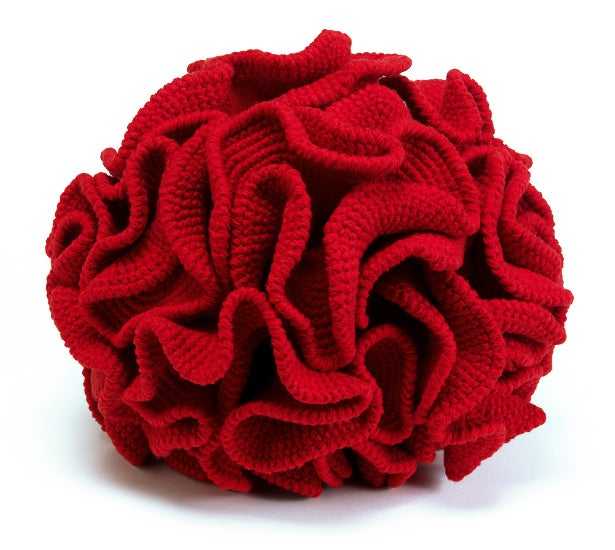# How Curvature Makes a Shape a Shape

The ancient study of an object’s curvature is guiding mathematicians toward a new understanding of simple equations.Explore

Mathematicians since the geometers of ancient Greece have defined geometric shapes by studying how the objects are curved. For simple shapes, like the sphere and the cube, curvature is straightforward. The curvature of a sphere is distributed evenly over its entire surface; on a cube, curvature is concentrated in equal parts at each of the eight evenly spaced vertices.

Mathematicians have other ways to define curvature for more complex shapes. First, imagine a flat surface. Intuitively you understand that it has no curvature (the surface is flat, after all). To see that mathematically, draw a circle around a point on that surface. That circle has an angle of 360 degrees.

Now imagine a cone jutting out of the surface like a mountain. Take that cone out, make a straight cut from the mountain top to the base, and lay the cone flat. You’ll see a sector of a circle—that is, a circle with a missing piece, like Pac-Man with his mouth open. The angle of the missing part of the circle is the curvature of the cone.

You can also get “negative” curvature with shapes like a horse’s saddle. In one direction (in line with the head and tail) it curves up, while in the other direction (perpendicular to the first) it curves down. If you were to cut it open and attempt to flatten it, as you did with the cone, it would overlap itself because it has too much angle. The surplus angle is the negative curvature of the saddle. Artisans take advantage of negative curvature in the quirky pursuit of “hyperbolic crocheting”—they add stitches into a flat yarn circle to create negative curvature and a warped, space-timey aesthetic.

Curvature is also intimately bound up with another essential feature of geometric shape: distance. To measure distance between points, you have to know the underlying object’s curvature. “If you’re trying to put a distance function on Colorado, one option is as the bird flies, and from a curvature point of view that would be essentially flat. If you’re measuring distance over the mountains, the way you would walk or drive, then you have really quite a lot of curvature and the distance would be greater,” explained Laura DeMarco, a mathematician at Northwestern University.

When mathematicians encounter new shapes, they explore their curvature and distance measurements. And that’s what DeMarco and Kathryn Lindsey are doing for a special set of fractal-derived shapes, as featured in my new article 3-D Fractals Offer Clues to Complex Systems.” The shapes they’re investigating arise naturally as three-dimensional incarnations of polynomial equations like f(x) = x2 – 1. Yet they’re so unfamiliar to mathematicians that at this point, they’re still hard to visualize. DeMarco and Lindsey hope to uncover the properties of these shapes and, by doing so, to gain new insight into the nature of polynomial equations.